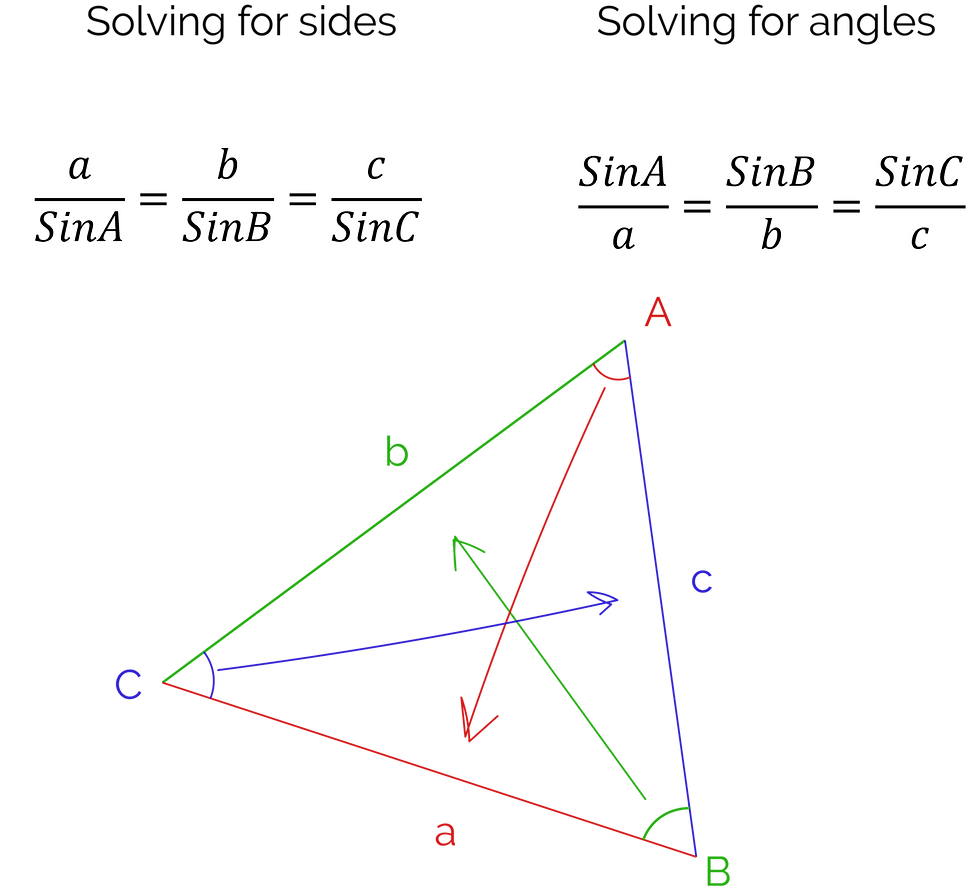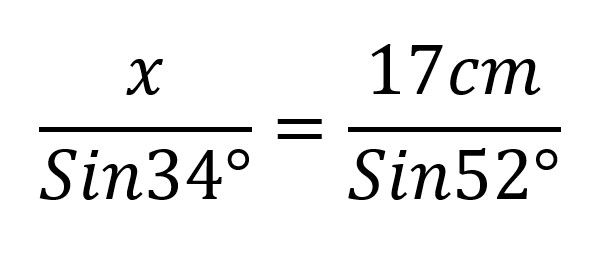cum
top of page
Search

# CSEC Mathematics: Sine and Cosine Rule

Sine and cosine rule both help us to find an unknown side or angle in a triangle. When you look at them, they seem quite daunting:But they're really not too difficult to deal with once you get the hang of them.

The interesting thing about these rules is that they apply to all triangles, including scalene triangles- unlike the trigonometric ratios (SOH CAH TOH) which only apply to right-angled triangles.

You'll notice that both of the rules are written in terms of a, b and c. So, first, you should understand how we label triangles:As you can see, the angle is named using a capital letter, and the side opposite to the angle is labelled with the common letter. So, an angle A has a side opposite to it named a.

This helps us to understand the laws a bit more.

Each rule has two ways of expressing it, one where the angle is easier to solve and one where the side is easier to solve.When solving for angles, the sine of the angles are on top, but when solving for sides, the sides are on top. You don't really need to use two different formulae, it just makes life easier.

Please also note that you will never have to use all three of the parts of the sine law, only two will ever be required.

.

We can use sine rule when we know three things: an angle, the opposite side, and the opposite side or angle of the side or angle we want to find.

So, if we're finding an angle, we need the length of the side opposite to it to use sine rule; and if we're finding a side, we need the size of the angle opposite to it, as well as the other two values.

Let's look at an example.Find x to 1 dp.

In this question, we can first label the values we know in terms of a, b and c.So, we know one angle and its opposite side (C and c) and the angle opposite of x, which is B. Therefore, we can use sine rule.

(Note: it doesn't matter how you label it, just so long as you know how to arrange your known values)

b= x, B= 34°, C= 52°, c=17 cm

Now, we can substitute these values into the sine rule formula:All we do now is transpose to find x.We can do the same thing for finding angles.Find the size of angle x to 1 dp.

We can label the triangle in terms of a, b and c (once again, this is completely arbitrary and doesn't have to be in any particular order, as long as the angle A is opposite to the side a, and so on).From this, we know an angle and a side opposite to it (C and c). We also know the length of the side opposite to x which is b.

We plug these values into the sine rule, where b=12cm, B=x, c=23cm and C=43°:Now, we can transpose so that we end up with Sin(x) on one side of the equation.Now that we have transposed, we have sin(x) on one side. To get the value of x, you would think to 'divide by sine,' but that isn't something that is technically possible. However, you can find the inverse of sine of the value on the other side of the equation. This gives us our value for x.

(You can find some sine rule practice questions here)

Let's look at cosine rule now.

Once again, cosine rule has two forms, one where the side is in the subject and the other where the angle is in the subject.As shown above, if you know two sides and the angle in between, you can use cosine rule to find the third side, and if you know all three sides, you can find the value of any of the angles in the triangle using cosine rule.

For example:Find x to 1 dp.

In cosine rule, it would be useful for you to name the unknown value as a (or A if it's an angle). This makes it so that you only have to remember two formulae, at most.Now, we can substitute the values into the cosine rule, where a=x, b=14 cm, c=17cm and A=54°With the values substituted, we just have to find the value of this monstrous looking expression.

Please note that the term at the end, -2(14)(17)Cos54, is found by multiplying -2 by 14 and then 17, and then multiplying that value by the cosine of 54°.We find the square root of both sides of the equation, and we get the length of x.

Now that we understand how to use cosine rule to find the length of a side given the requisite information, let's take a look at finding angles with cosine rule.Find x to 1 d.p.

Let's start the same way we did last time: label our unknown angle using A, and label the rest of the triangle correspondingly.Now, we can substitute the values into the cosine rule for angles, where A=x, a=34cm, b=21cm, c=13cm.We simply find the value of this expression and then find the cosine inverse of that.Please note that this triangle cannot actually exist.

(Find some practice questions on cosine rule here)